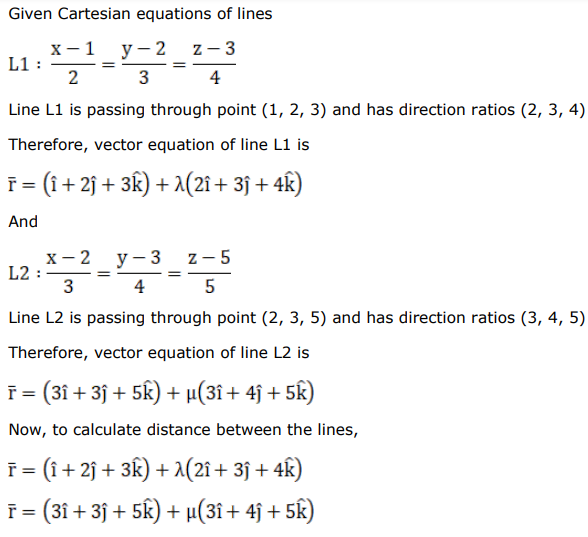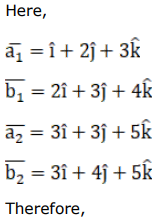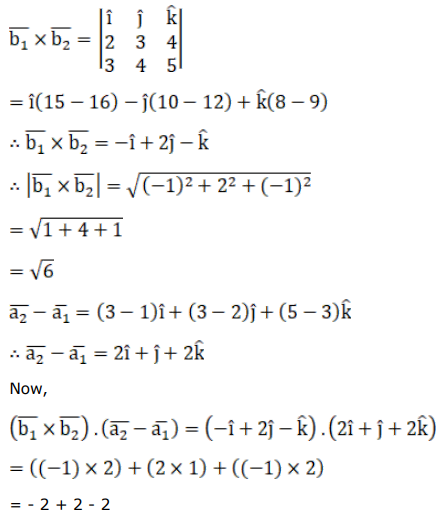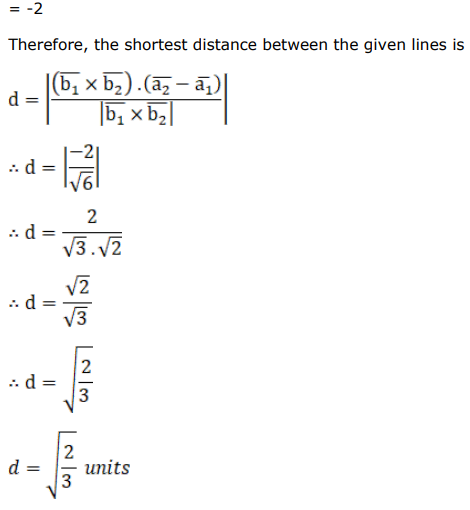# Write the vector equation of the following lines and hence find the shortest distance between them :Question:

Write the vector equation of the following lines and hence find the shortest distance between them :

$\frac{x-1}{2}=\frac{y-2}{3}=\frac{z-3}{4}$ and $\frac{x-2}{3}=\frac{y-3}{4}=\frac{z-5}{5}$.

Solution: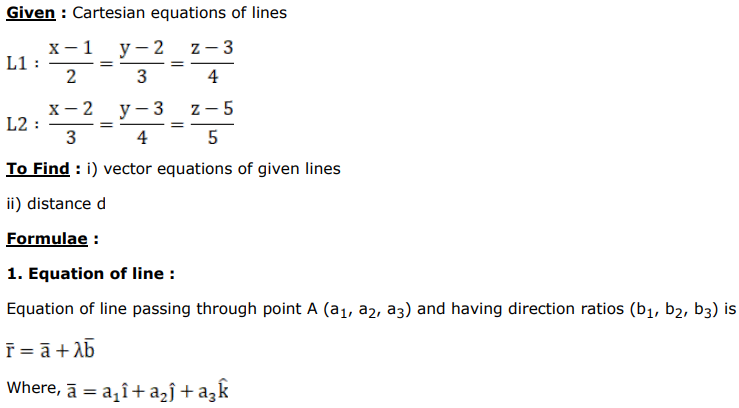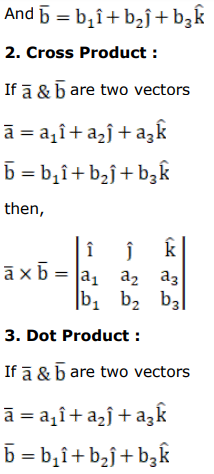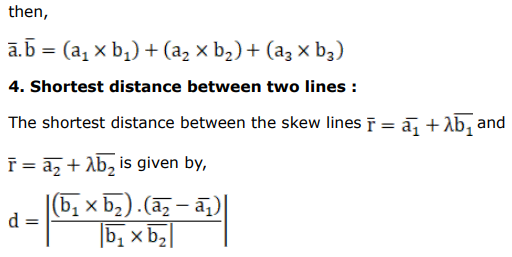.